# Getting Started with D3.js

## What is D3?

• Data-Driven Documents
• Powerful and flexible JavaScript library
• Leverages HTML, SVG and CSS
• Examples and docs at http://d3js.org/

## Basically...It's the sort of library that showcases what JavaScript can do when approached properly.

## Parking Ticket Data?

• ~2.8 million parking tickets/year in Toronto
• Includes date, time, infraction, fine amount, etc.
• Available from Toronto Open Data
• This presentation uses the 2012 data

## can be daunting:

• Lots and lots of methods
• Each methods does a very specific task
• Most methods are chainable
• Visualizations need to be built up piecemeal

## But Don't Panic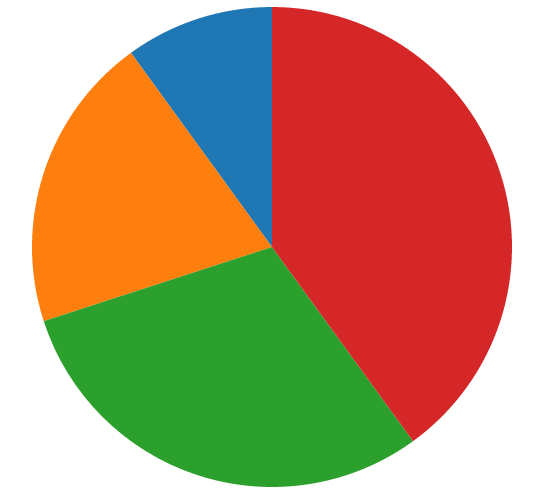### Looking at the DOM: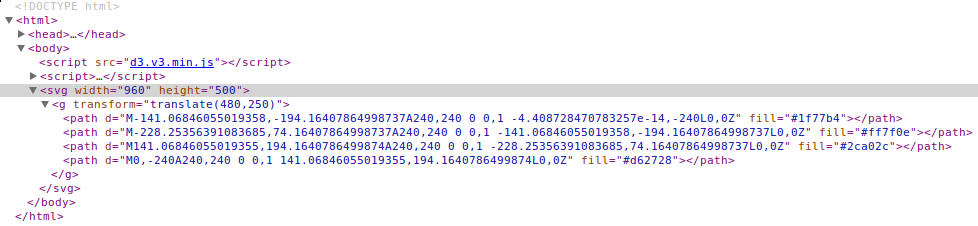• An svg element with a width and height
• A g element that has been translated to center it
• 4 path elements with d and fill attributes

## So how do we do that?

`` var data = [10, 20, 30, 40];``

Then we'll define the dimensions:
`````` var width = 960,
height = 500,
radius = Math.min(width, height) / 2;``````

## So far so good...

Next we'll define the colours  using
a built-in D3 palette:
`` var color = d3.scale.category10();``
Other palettes are available and you can
always just specify your own colours.

## Now things get interesting:

Because pie charts are circular,
we'll need to define an arc:
`````` var arc = d3.svg.arc()
This is where we specify the radius of the chart.

We'll extend this later on but this is a good start.

## What would a pie chart be without a pie variable?

`````` var pie = d3.layout.pie()
.value(function(d) { return d; });``````
This calculates the angles for each section.
In other words, it determines the size of each wedge.

The value method takes an accessor function
that returns the value for each data point.
For our simple data, we can pass in an identity function.

## Now for the SVG:

`````` var svg = d3.select('body')
.append('svg')
.attr('width', width)
.attr('height', height)
.append('g')
.attr('transform', 'translate(' + width / 2 + ',' + height / 2 + ')');``````
This may look complicated but all it's doing is:
• setting the width and height on it
• translating it so that it's centered

## Let's compare:

`````` var svg = d3.select('body')
.append('svg')
.attr('width', width)
.attr('height', height)
.append('g')
.attr('transform', 'translate(' + width / 2 + ',' + height / 2 + ')');``````## No problem, right?## And finally:

`````` var path = svg.selectAll('path')
.data(pie(data))
.enter()
.append('path')
.attr('d', arc)
.attr('fill', function(d, i) { return color(i); });``````
Here we see the majestic D3 pattern
selectAll(...).data(...).enter.()..append(...)

I know what you're thinking...## take it line by line:

`````` // Select the nodes to which we'll bind the data
var path = svg.selectAll('path')

// Bind the pie-ified data to the selection
.data(pie(data))

// Create placeholder nodes for each data point
.enter()

// Append the path elements to the svg
.append('path')

// Provide the arc data for each data point
.attr('d', arc)

// Pick a colour from the palette by iterating over the data points
.attr('fill', function(d, i) { return color(i); });
``````

## It's a lot of steps but D3's flexibility stems from its use of small, chainable methods.

You'll come across variations of the
selectAll(...).data(...).enter().append(...)
pattern again and again in D3.

## The finished product

The complete code can be seen on GitHub.

A live example can be found on S3.

Great, but....

## What was all that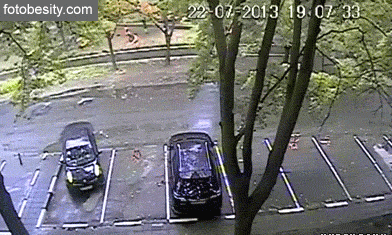## Phase 2

Let's take this a bit further by doing two things:
1. Using real data instead of our dummy data
2. Switching to a donut chart

## We'll end up with this:## The donut part is easy## It's a one-line change:

Simply take this:
`````` var arc = d3.svg.arc()
`````` var arc = d3.svg.arc()
innerRadius(...) defines where the segment starts
while outerRadius(...) defines where it stops.

## Now for some real data:

`````` province,count
ON,2612317
Other,46623
QC,36620
AB,12267
NY,10919
BC,7019
NS,6008
FL,5464
MI,5008
MB,3909``````
This breaks down the total number of issued tickets by
province/state. Only the top nine are listed explicitly
with all others combined in the Other category.

This was extracted from the parking ticket data using
Python and Pandas (which is awesome, by the way).

(as well as TSVs and arbitrary DSVs)
Our province/state data will be transformed into:
`````` [{
province: "ON",
count: 2612317``````  },
{
province: "Other",
count: 46623
},
{
province: "QC",
count: 36620
},``````  ...
``````
Note that the column headers have become
object keys.

## D3.csv(...) is asynchronous

Therefore we need to move the parts that rely
on the data into a callback. In our case, that means
our friend selectAll(...).data(...).enter().append(...)
`````` d3.csv('provinces.csv', function(error, data) {
data.forEach(function(d) {
d.count = +d.count;
});

var path = svg.selectAll('path')
.data(pie(data))
.enter()
.append('path')
.attr('d', arc)
.attr('fill', function(d, i) { return color(i); });
});``````
Note that the var path = ... part is unchanged.

## CSVs fields are strings

Which is why we see the following on the preceding slide:
`````` data.forEach(function(d) {
d.count = +d.count;
});``````
This is another pattern you'll see a lot in D3 examples.

We need the count field to be a number, so we could use parseInt(...) or parseFloat(...) but using the + to cast it to a number is generally faster. (The joys of JS.)

## We now have an array of objects instead of an array of numbers

So there's one other change we need to make. This:
`````` var pie = d3.layout.pie()
.value(function(d) { return d; });``````
Becomes:
`````` var pie = d3.layout.pie()
.value(function(d) { return d.count; });``````
Now we see how the value accessor function works: it needs to extract a numeric value from the object.

## Phase 2 is complete.

Code: GitHub

Example: S3

That means it's time for...

## Phase 3

Our chart is looking pretty good but
what does each colour represent?

## But not this kind:## More like this: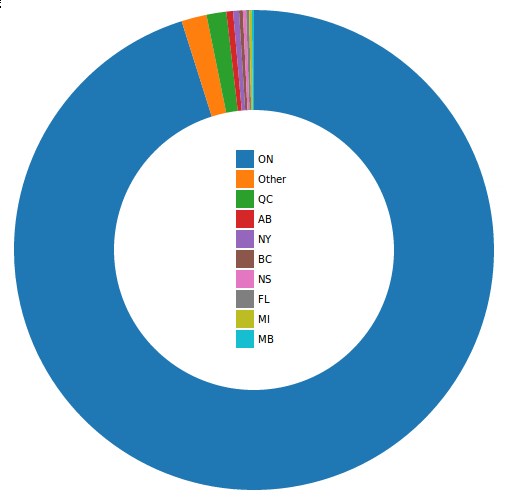## Our first CSS

So far we've gotten along without any CSS at all.
(Take a moment to consider that.)

Now we'll add just a little bit to make the font nicer:
`````` body {
font: 10px sans-serif;
}``````

## Now what?

Up until now we've been specifying the
colours using the data indices:
`````` var path = svg.selectAll('path')
... // Other methods
.attr('fill', function(d, i) { return color(i); });``````
We're going to switch to using the province/state name:
`````` var path = svg.selectAll('path')
... // Other methods
.attr('fill', function(d, i) { return color(d.data.province); });``````
This will create an association between
the two that we'll use shortly.

## Our legend needs three parts

1. A wrapper
2. The coloured boxes
3. The text (province/state name)

## The Wrapper

In the CSV callback, after var path = ... we'll add:
`````` var legend = svg.selectAll('.legend')
.data(color.domain())
.enter()
.append('g')
.attr('class', 'legend')
.attr('transform', function(d, i) {
return 'translate(0,' + (i * 20 - 100) + ')';
});
``````
You should recognize the selectAll(...).data(...).enter().append(...)
pattern, after which we just add a legend class
and use a transform to position the wrapper
in the center of the donut.

## The Coloured Boxes

These are straightforward:
`````` legend.append('rect')
.attr('width', 18)
.attr('height', 18)
.attr('x', -18)
.style('fill', color);
``````
This adds an 18x18 rectangle for each data point,
offsets them for centering purposes
and finally specifies the fill colour.

## The Text

This is also straightforward:
`````` legend.append('text')
.attr('x', 4)
.attr('y', 9)
.text(function(d) { return d; });``````
This adds a text element for each data point,
positions it accordingly and finally defines the text,
which will be the province/state name due to the
association we created earlier.

## Easy mode, right?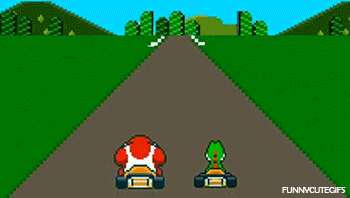Code: GitHub

Example: S3

Onwards we go...

## Time for some tooltips:## A little more CSS

Tooltips aren't built into D3 (although
there are extensions you can use), so
we need some more styles:
`````` .tooltip {
background: #eee;
color: #333;
position: absolute;
text-align: center;
visibility: hidden;
z-index: 10;
}``````

## .on() and mouse events

We'll use three event handlers to enable our tooltips.
They will be added to path in the callback:
`````` path.on('mouseover', tooltipOn)
.on('mousemove', tooltipMove)
.on('mouseout', tooltipOff);``````

## Before we do anything else...

We need to add a tooltip to the document.
This doesn't need to be in the callback: it can
go after the var pie = ... part.
`````` var tooltip = d3.select('body')
.append('div')
.attr('class', 'tooltip');``````
This just adds a div to the body and
gives it the tooltip class.

## The 'mouseover' handler

Next we add our handlers, starting with tooltipOn:
`````` var tooltipOn = function(d, i) {
var content = '<div>' + d.data.province + '</div><div>' +
d.data.count + '</div>';
tooltip.html(content)
.style('visibility', 'visible');
};
``````
When the mouse hovers over a segment,
our tooltip will update its content to include
the province/state name and the count.

It will also make the tooltip visible
(which is kind of important).

## The 'mousemove' handler

We'll have the tooltip move with the mouse, which means it needs to know where the cursor currently is:
`````` var tooltipMove = function(d, i) {
tooltip.style('top', (d3.event.pageY + 10) + 'px')
.style('left', (d3.event.pageX + 10) + 'px');
};``````
We can get the position from d3.event.
The + 10 is there to provide some offset.

## The 'mouseout' handler

This is the simplest of the three, all it needs to do is hide the tooltip when the mouse is no longer over a segment:
`````` var tooltipOff = function() {
tooltip.style('visibility', 'hidden');
};``````

With that, our tooltip is good to go.

## Give it a tryCode: GitHub

Example: S3

Now just one last thing...

## Phase 5

Let's get things moving...## One of the most exciting aspects of D3 is its ability to enable interactivity.

Our chart is currently dominated by the parking tickets from Ontario, so it's hard to get a good feel for the breakdown of the other provinces/states.

## Let's toggle between this:## And this: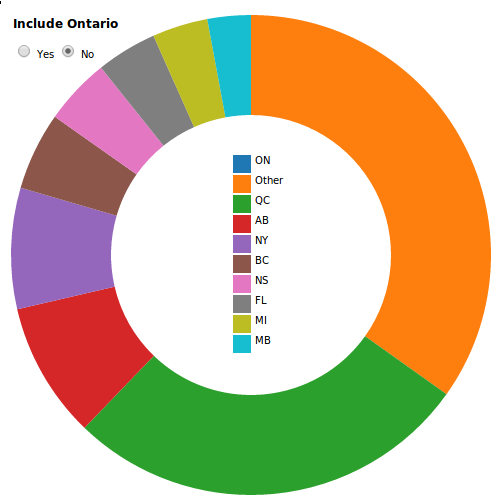## First we'll add our form

We'll put this at the start of the body:
`````` <form>
<h3>Include Ontario:</h3>
<label><input type="radio" name="ontario" value="yes" checked> Yes</label>
</form>
``````
And add a bit of CSS:
`````` form {
left: 250px;
position: absolute;
}
label {
cursor: pointer;
}``````

## So how will we do this?

We're going to register event listener on input elements:
`` d3.selectAll('input').on('change', change);``

## Now for the logic

`````` var change = function() {
if (this.value === 'yes') {
pie.value(function(d) { return d.count; });
} else {
pie.value(function(d) {
return (d.province === 'ON') ? 0 : d.count;
});
}
path = path.data(pie);
path.transition().duration(750).attrTween('d', arcTween);
};
``````
this refers to the input that was just changed.
If its value is yes, we proceed as before.
If its value is no, we'll set the Ontario count to 0.
We then need to recalculate the angles
and finally we'll animate the transition.

## Before we discuss the animation, there is some housekeeping to be done.## Disable sorting:

`````` var pie = d3.layout.pie()
.value(function(d) { return d.count; })
.sort(null); // NEW``````

This isn't strictly necessary but the animation
will look  a bit wonky otherwise.

## Separate out the data

This:
`````` var path = svg.selectAll('path')
.data(pie(data))
.enter()
.append('path')
.attr('d', arc)
.attr('fill', function(d, i) { return color(d.data.province); });``````
Becomes:
`````` var path = svg.datum(data) //NEW
.selectAll('path')
.data(pie) // CHANGED
.enter()
.append('path')
.attr('d', arc)
.attr('fill', function(d, i) { return color(d.data.province); })
.each(function(d) { this._current = d; });  // NEW
``````

## going on here:

1. Moving data into .datum() keeps it from
being joined with the selection, which is
what happens with .data().
2. The last line attaches a _current value to
each data point. This will be used in the
animation.

## Finally we have arcTween

I yanked this straight out of a D3 animation example:
`````` var arcTween = function(a) {
var i = d3.interpolate(this._current, a);
this._current = i(0);
return function(t) {
return arc(i(t));
};
};``````
The attrTween method allows D3 to transition smoothly between two values. In this case we're providing arc values.## Our pie chart is done.

Code: GitHub
Example: S3